Function Repository Resource:

# NeumannO

Evaluate the Neumann polynomial

Contributed by: Yury Brychkov and Jan Mangaldan
 ResourceFunction["NeumannO"][n,z] gives the Neumann polynomial On(z) .

## Details

Mathematical function, suitable for both symbolic and numerical manipulation.
The Neumann polynomial is defined as:Neumann polynomials satisfy the generalized generating function relation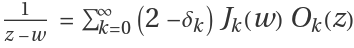.
Neumann polynomials satisfy the integral representation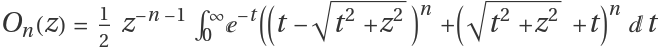.
Neumann polynomials satisfy the differential equation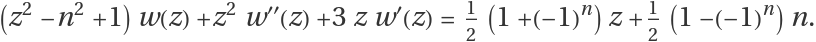Neumann polynomials are rational functions and not strictly polynomials.
ResourceFunction["NeumannO"] can be evaluated to arbitrary numerical precision.

## Examples

### Basic Examples (3)

Evaluate numerically:

 In:=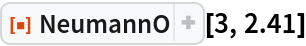Out=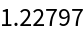Evaluate Neumann polynomials for various orders:

 In:=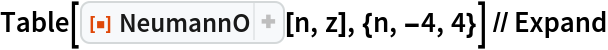Out=Plot with respect to z:

 In:=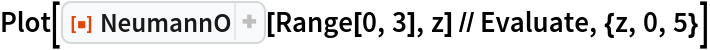Out=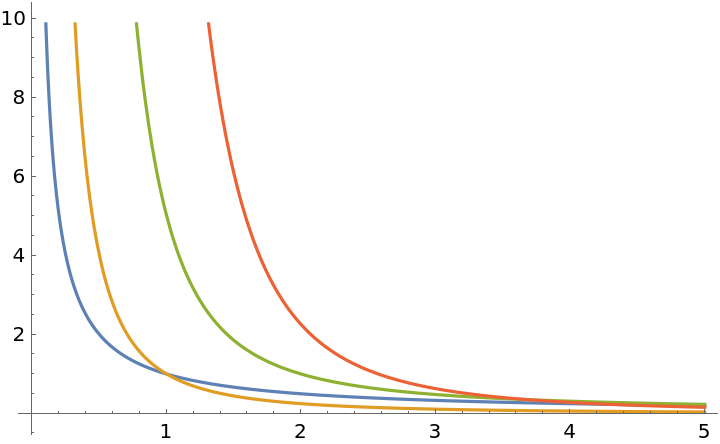### Scope (3)

Evaluate for complex arguments:

 In:=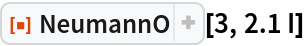Out=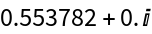Evaluate to high precision:

 In:=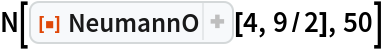Out=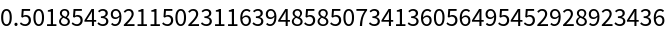The precision of the output tracks the precision of the input:

 In:=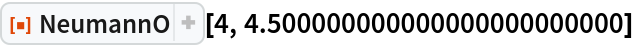Out=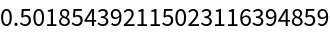In:=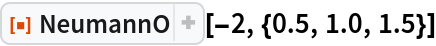Out=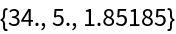### Applications (3)

Define a function: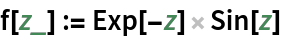Use NeumannO to expand a function in a Bessel function series:

 In:=Out=Compare the function with its Bessel series approximation:

 In:=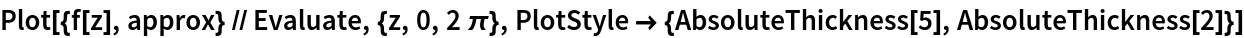Out=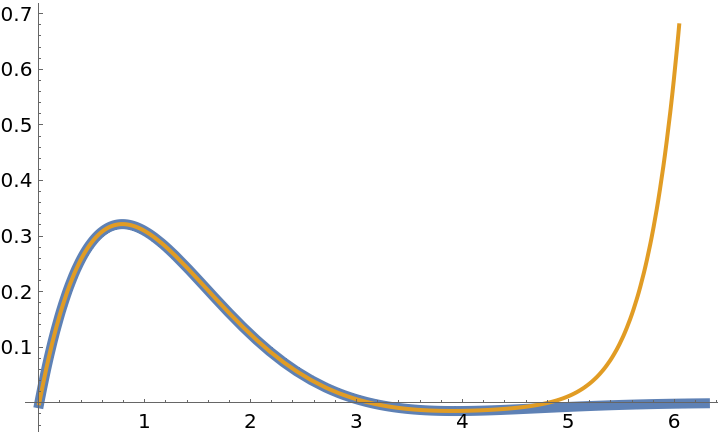### Properties and Relations (8)

Derivatives of Neumann polynomials are related to the polynomials themselves via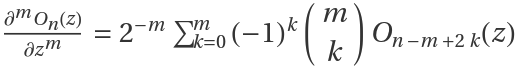:

 In:=Out=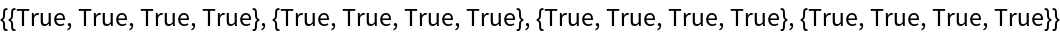Neumann polynomials satisfy the differential equation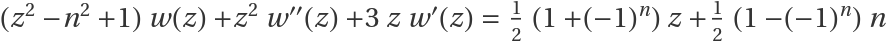:

 In:=Out=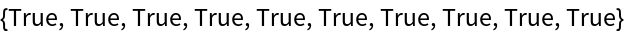Neumann polynomials satisfy the recurrence identity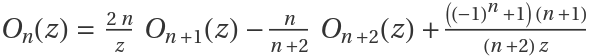:

 In:=Out=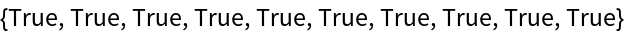The Neumann polynomials have the limiting behavior given by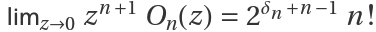:

 In:=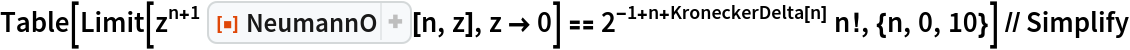Out=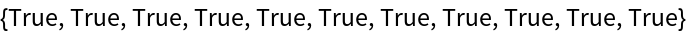Neumann polynomials can be represented as the finite sum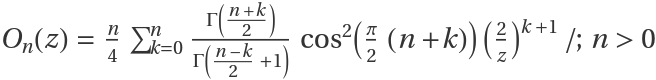:

 In:=Out=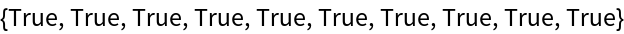The Neumann polynomials can be expressed in terms of HypergeometricPFQ through the formula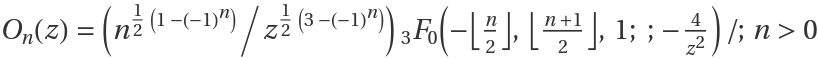:

 In:=Out=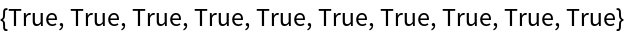Neumann polynomials can be expressed in terms of the Lommel function:

 In:=Out=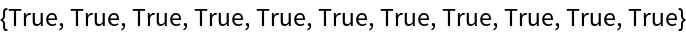Neumann polynomials can be expressed in terms of the Schläfli polynomial:

 In:=Out=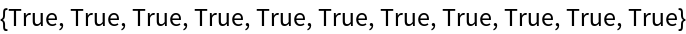## Version History

• 1.0.1 – 31 August 2021
• 1.0.0 – 06 December 2019